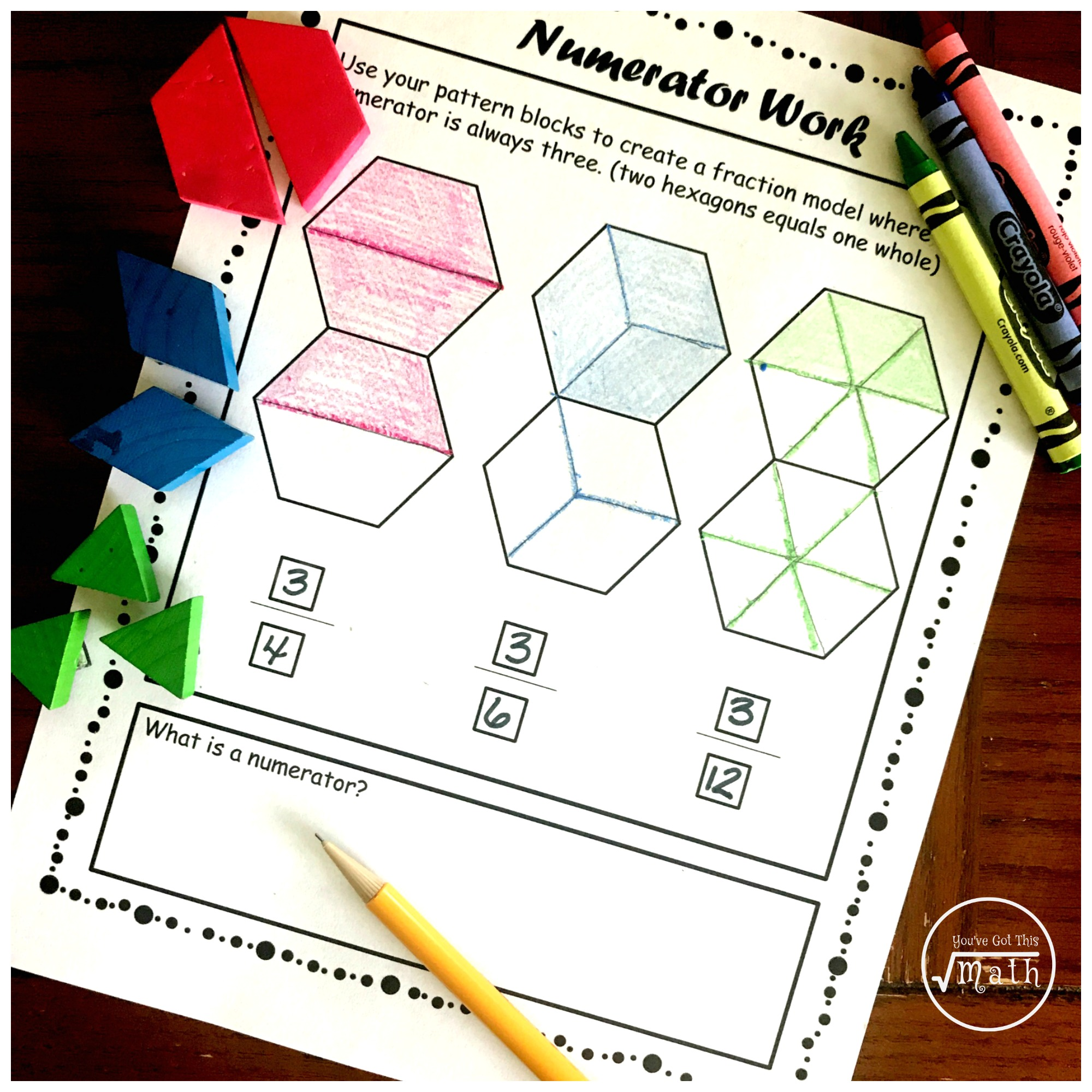Home » Math » Grab These Free Fraction Of A Group Word Problems

Grab These Free Fraction Of A Group Word Problems

These Fraction of a Group Word Problems are a great way for children to analyze and understand fractions when they are presented as parts of a set.

The free printable can be found at the END of this post. Click on the blue “get your free printable here” button.

It doesn’t happen very often, but periodically I find a math concept that stumps my seven-year-old. For the most part, he just flies through math, whipping out answers, and surprising his mom with his number sense.

This fraction concept puzzled him. I could see a frustration in his eyes, that normally only appears during writing.

What was causing him such anguish? A fraction of set word problems.

Fraction of a Set

So often most of our fractional instruction revolves around fractions being apart of a whole. We focus on pies, pizza, and visual models. But, we leave out the fact that fractions can be used to be part of a set….such as 2/9 of the M&M’s in the pack are red.

We had played games with this concept, done the clip cards from this fraction set, and had worked on it in through some worksheets in our math curriculum. But now that it was time for some word problems….we were stuck.

What is Fraction of a Set Word Problems

There are two main type of fractions of a set word problems. The first type gives children information about the part, and then the children have to figure out what the whole is. The other one will tell you information about the whole, and the children will have to figure out about the part.

What is the Whole?

An example of this type of problem might be…..

2/3 are blue, and there are 10 blue shirts.

The children begin by taking the number and dividing it up by the numerator. 10 ÷2 = 5

Now they know that there are 5 shirts in each group. By looking at the denominator they know that there are 3 groups. Soooo, they multiply 5 x 3 which equals 15. There are 15 shirts!!!

What is the Part?

When solving a problem like this, you might see…..

2/5 of the shirts are blue. If there are 15 shirts, how many are blue?

First, the children should figure out how many shirts are in each group. So they would take the 15 shirts and divide them into 5 (denominator) equal groups. 15 ÷ 5 = 3

They now know that there are 3 shirts in each group. Since two of the groups (numerator) have blue shirts, the children multiply 3 x 2 to find out that 6 of the shirts are blue.

Prep – Work

There is very little prep work for these printables.

1. First, print off the sheets.
2. Next, provide counting bears, colored pencils, and a pencil.
3. Finally, gather up your 3rd through fifth graders and you are ready to go.

How to Use

First, have the children read the problem, and underline important information.

In the example above, the student would want to know that 3/5 of the bears are blue, the rest are green, and that there are 15 altogether.

Next, the students use the colored bears to work out the problem.

Using the problem above, the children would count out 15 blue bears. Next, they would divide them into five equal groups since the denominator tells us there are five groups.

15 ÷ 5 = 3 so we know that there are three bears in each group.

Using the bears in front of them, they write the answer to the problem in the answer box.

Three of the groups are blue so 3 x 3 = 9. Nine of the shirts are blue.

Two groups are green, so 3 x 2 = 6. Nine of the shirts are green.

Finally, the children write out an equation for what they did.

The children started off taking the 15 bears and dividing them into three groups. They then skip counted or multiplied to figure out how many bears there were altogether.

15 ÷ 5 = 3

2 x  3 = 6 or 3 x 3 = 9.

As we worked through these problems, I began to see the frustration begin to dissolve.  So we get to move unto our next topic. Two-Digit multiplication!!!!

You’ve Got This

Rachel

You may also be interested in:

A review on denominators and numerators.More fraction of a Group Word Problems using Gum Drops. This packet also has multiplying fractions, measuring, line graphs, STEAM and more.